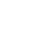Introducing Visual Explorer, a new tool for data visualization.Learn more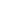# Creating Histograms using Pandas

A histogram is a graphical representation commonly used to visualize the distribution of numerical data. When exploring a dataset, you'll often want to get a quick understanding of the distribution of certain numerical variables within it. You can do this by using a histogram. A histogram divides the values within a numerical variable into “bins”, and counts the number of observations that fall into each bin. By visualizing these binned counts in a columnar fashion, we can obtain a very immediate and intuitive sense of the distribution of values within a variable.

This recipe will show you how to go about creating a histogram using Python. Specifically, you’ll be using pandas `hist()` method, which is simply a wrapper for the matplotlib pyplot API.

In our example, you're going to be visualizing the distribution of session duration for a website. The steps in this recipe are divided into the following sections:

You can find implementations of all of the steps outlined below in this example Mode report. Let’s get started.

## Data Wrangling

You’ll use SQL to wrangle the data you’ll need for our analysis. For this example, you’ll be using the `sessions` dataset available in Mode's Public Data Warehouse. Using the schema browser within the editor, make sure your data source is set to the Mode Public Warehouse data source and run the following query to wrangle your data:

``````select *
from modeanalytics.sessions
``````

Once the SQL query has completed running, rename your SQL query to `Sessions` so that you can identify it within the Python notebook. You can do this by navigating to the 3 dots next to ‘Query 1” in your editor toolbar and clicking “Rename.”

## Data Exploration & Preparation

Now that you have your data wrangled, you’re ready to move over to the Python notebook to prepare your data for visualization. Inside of the Python notebook, let’s start by importing the Python modules that you'll be using throughout the remainder of this recipe:

``````import numpy as np
import pandas as pd
import matplotlib.pyplot as plt
from matplotlib.ticker import StrMethodFormatter
``````

Mode automatically pipes the results of your SQL queries into a pandas dataframe assigned to the variable `datasets`. You can use the following line of Python to access the results of your SQL query as a dataframe and assign them to a new variable:

``````df = datasets['Sessions']
``````

You can get a sense of the shape of your dataset using the dataframe `shape` attribute:

``````df.shape
``````

Calling the `shape` attribute of a dataframe will return a tuple containing the dimensions (rows x columns) of a dataframe. In our example, you can see that the sessions dataset we are working with is 65,499 rows (sessions) by 5 columns. You can investigate the data types of the variables within your dataset by calling the `dtypes` attribute:

``````df.dtypes
``````

Calling the `dtypes` attribute of a dataframe will return information about the data types of the individual variables within the dataframe. In our example, you can see that pandas correctly inferred the data types of certain variables, but left a few as `object` data types. You have the ability to manually cast these variables to more appropriate data types:

``````# Data type conversions
df['created_at'] = df['created_at'].astype('datetime64[ns]')
df['user_type'] = df['user_type'].astype('category')

# Show new data types
df.dtypes
``````

Now that you have your dataset prepared, we are ready to visualize the data.

## Data Visualization

### Pandas hist()

To create a histogram, we will use pandas `hist()` method. Calling the `hist()` method on a pandas dataframe will return histograms for all non-nuisance series in the dataframe: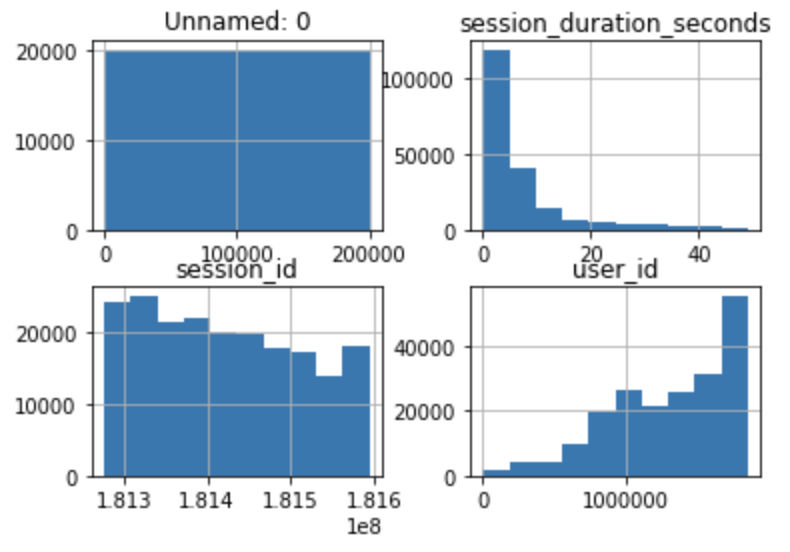### Key Histogram parameters

Most of the time, when visualizing data, you want to highlight specific variables. To do this using pandas histograms, you would need to utilize it’s parameters. Below are some of the most common pandas `hist()` parameters:

• column: the specific column(s) you want to create a histogram of
• by: the parameter on which to split your data; this produces multiple histograms displaying each group
• bins the number of buckets your data will be grouped by (the number of bars in your histogram); pandas automatically creates bins if not specified

Since you are only interested in visualizing the distribution of the `session_duration_seconds` variable, you will pass in the column name to the `column` argument of the `hist()` method to limit the visualization output to the variable of interest:

``````df.hist(column='session_duration_seconds')
``````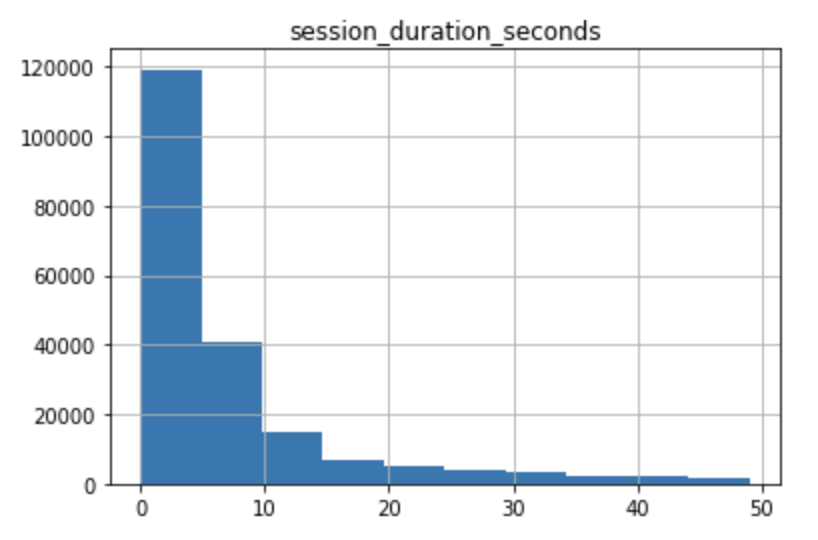You can further customize the appearance of your histogram by supplying the `hist()` method additional parameters and leveraging matplotlib styling functionality:

``````ax = df.hist(column='session_duration_seconds', bins=25, grid=False, figsize=(12,8), color='#86bf91', zorder=2, rwidth=0.9)

ax = ax
for x in ax:

# Despine
x.spines['right'].set_visible(False)
x.spines['top'].set_visible(False)
x.spines['left'].set_visible(False)

# Switch off ticks
x.tick_params(axis="both", which="both", bottom="off", top="off", labelbottom="on", left="off", right="off", labelleft="on")

# Draw horizontal axis lines
vals = x.get_yticks()
for tick in vals:
x.axhline(y=tick, linestyle='dashed', alpha=0.4, color='#eeeeee', zorder=1)

# Remove title
x.set_title("")

# Set x-axis label
x.set_xlabel("Session Duration (Seconds)", labelpad=20, weight='bold', size=12)

# Set y-axis label

# Format y-axis label
x.yaxis.set_major_formatter(StrMethodFormatter('{x:,g}'))
``````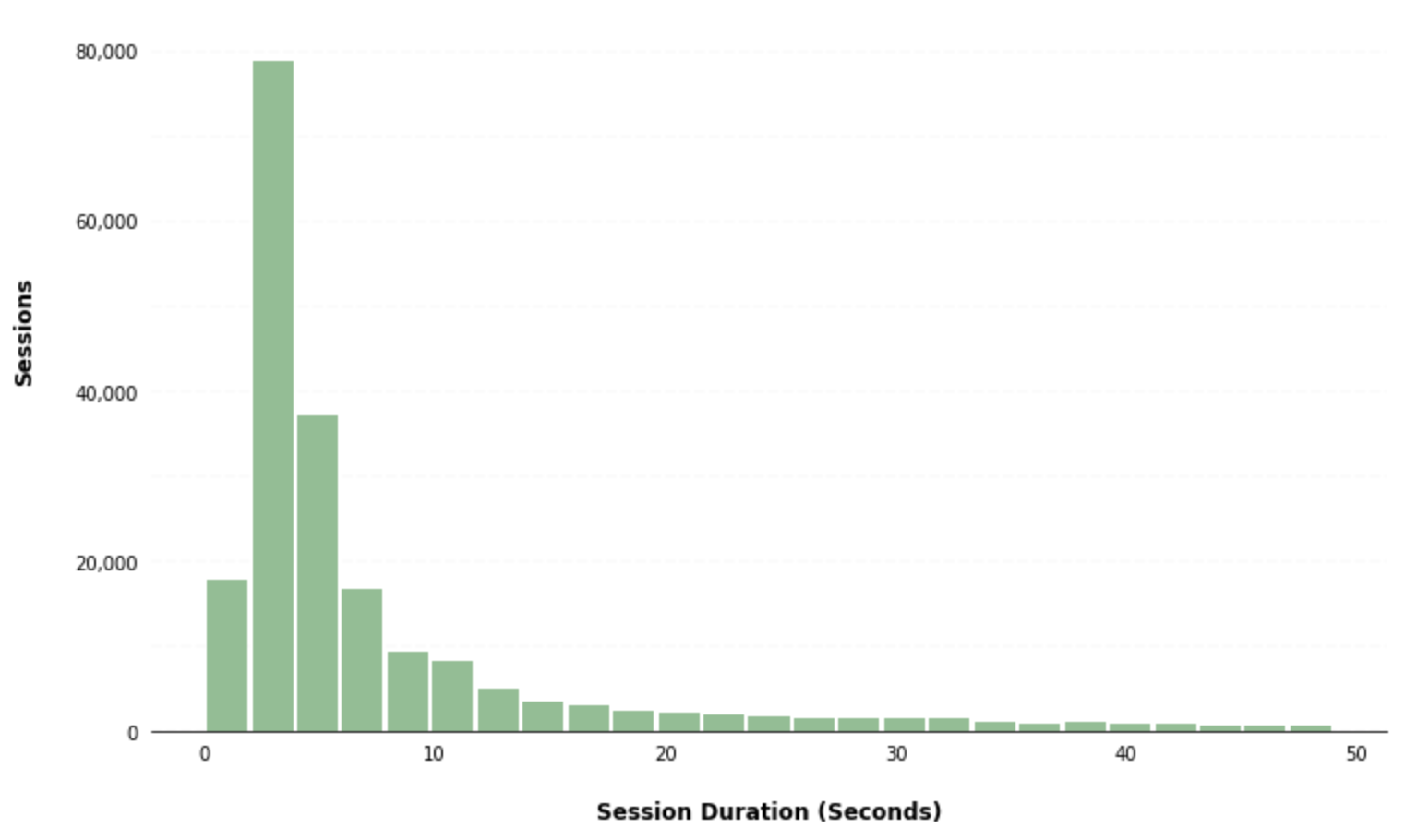The pandas `hist()` method also gives you the ability to create separate subplots for different groups of data by passing a column to the `by` parameter. As an example, you can create separate histograms for different user types by passing the `user_type` column to the `by` parameter within the `hist()` method:

``````ax = df.hist(column='session_duration_seconds', by='user_type', bins=25, grid=False, figsize=(8,10), layout=(3,1), sharex=True, color='#86bf91', zorder=2, rwidth=0.9)

for i,x in enumerate(ax):

# Despine
x.spines['right'].set_visible(False)
x.spines['top'].set_visible(False)
x.spines['left'].set_visible(False)

# Switch off ticks
x.tick_params(axis="both", which="both", bottom="off", top="off", labelbottom="on", left="off", right="off", labelleft="on")

# Draw horizontal axis lines
vals = x.get_yticks()
for tick in vals:
x.axhline(y=tick, linestyle='dashed', alpha=0.4, color='#eeeeee', zorder=1)

# Set x-axis label
x.set_xlabel("Session Duration (Seconds)", labelpad=20, weight='bold', size=12)

# Set y-axis label
if i == 1:

# Format y-axis label
x.yaxis.set_major_formatter(StrMethodFormatter('{x:,g}'))

x.tick_params(axis='x', rotation=0)
``````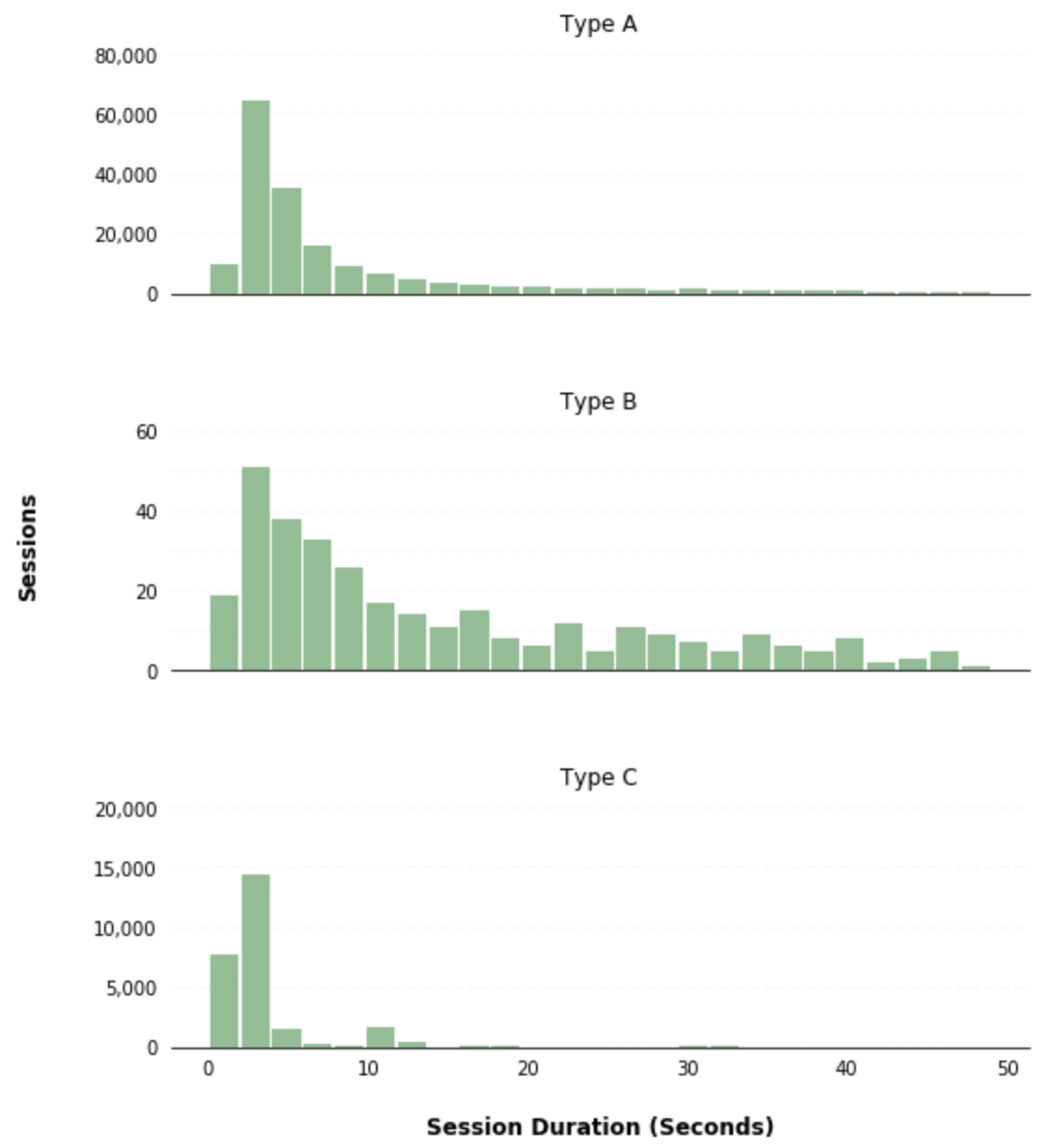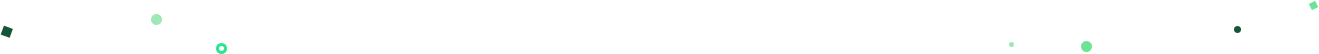# Get our weekly data newsletter

Work-related distractions for every data enthusiast.